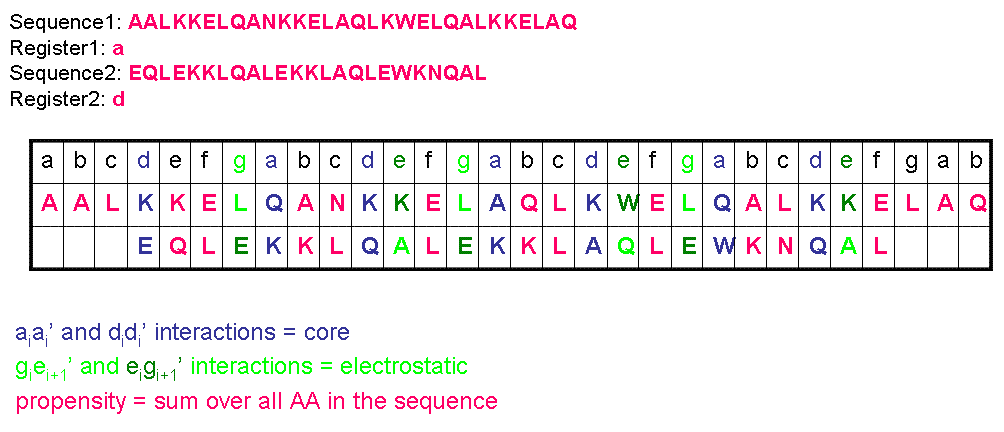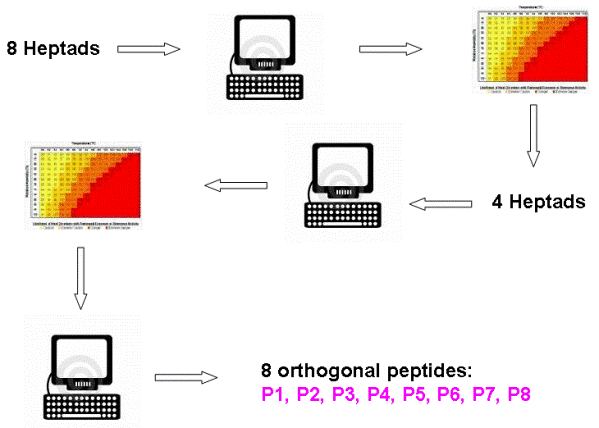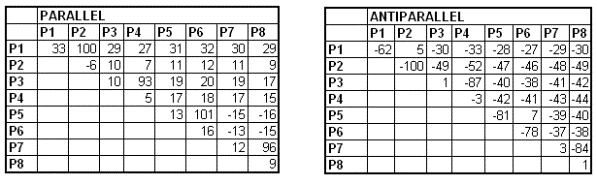# Team:Slovenia/Orthogonal coiled-coils.html

Modelling

## De novo design of orthogonal coiled-coil forming segments

One of the main challenges we were faced with was to ensure exclusivity of the pairing. Our selected heterodimeric coiled-coil-forming segments had to satisfy the following requirements:

• any coiled-coil-forming segment must form coiled-coil with exactly one segment,
• any other combination is forbidden/significantly disfavored.

In this way selected system of coiled-coil forming segments is called orthogonal.
Additionally we have to ensure that selected proteins are not too long (in order to avoid bending as well as decreased energetic difference between desired and undesired pairs) but still have approximately the same length to form symmetrical structures if not otherwise required by some special design.
As mentioned in the introduction, coiled-coils can be parallel or antiparallel as well as homodimers and heterodimers. These are also features we have to consider when planning (i.e. we identify a parallel pair but have to also check that neither of proteins forms more stable parallel/antiparallel homodimer/heterodimer).

Based on the published rules for the prediction of stability of coiled-coils (Hageman et al., 2008) we prepared a program to predict the stability of each tested pair.

In order to restrict the number of possible combinations to manageable we limited the variability at defined positions to a limited set of residues that have been previously shown to stabilize the interactions. We started by choosing the most appropriate amino acids for the every position in sequence, similar as reported (Bromley et al., 2009). Generally we followed the rule that hydrophobic residues at positions “a” or “d” and opposite charge at positions “e” and “g” on the equivalent positions between the two helices stabilize, whereas burial of polar residues (Asn) at positions “a” or “d” and the same charge at positions “e” and “g” destabilize the structure (see Introduction). In this simplified model we considered a limited number of variable residues only at positions that significantly affect the stability (“a”, “d”, “e”, “g”) and neglecting the effect on other positions (positions “b”, “c”, “f”). It has to be noted that the parameters to predict stability have been extracted from parallel dimers of basic leucine zipper (bZIP) peptides, therefore the predictions for this type of interaction are most reliable. Although we can probably estimate also contributions to the stability of antiparallel orientation, we tried to use experimentally parallel orientations whenever it was possible.

To predict coiled-coil interactions we examined two algorithms (see Hagemann et al., 2009; Fong et al., 2006) and in the end chose to follow Hageman et al.
There are many factors that affect stability: hydrophobic burial, propensity, solubility, electrostatic interaction of flanking residues and others. This algorithm considers three factors: core, electrostatic and propensity which seem to have the key role in CC formation.
The calculation is shown in following scheme (shown on a fictional protein pair) (Figure 1).Figure 1

We extended this algorithm to pairs with different lenghts and register as well as antiparallel peptides.
Complete algorithm (in C, easily applicable to C++/Matlab/Mathematica): link and in Excel (just for pairs of with set register): link

## Identification of a set of orthogonal coiled-coil pairsFigure 2

Figure 2 above shows our approach:

First we calculated the interaction energy between all possible pairs of 8 different heptads. The interaction of coiled-coil segment is additive, therefore the interaction between larger coiled-coil segments, composed of several heptads could be deduced from the interacting contributions of all heptads. Next we generated a table where we calculated the interaction energy between all possible combinations of coiled-coil-forming segments composed of four heptads in both parallel and antiparallel orientation.

Then we prepared another algorithm (download and abstract follow link) to pick the best set of orthogonal pairs from the generated table which was selected to maximize the difference between the least stable desired pair and most stable undesired pair in the selected set. Predicted temperature difference between the least stable desired pair and most stable undesired pair was predicted to be more than 70 °C.

As a result of this approach we found a system of 8 orthogonal designed peptides: P1, P2, P3, P4, P5, P6, P7 and P8, which form four pairs: P1+P2, P3+P4, P5+P6 and P7+P8.
In the following chart we can see theoretically predicted melting temperatures (higher the temperature, the more stable the coiled-coil).Figure 3